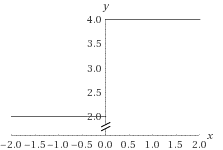# The domain and range of the function and sketch the graph.### Single Variable Calculus: Concepts...

4th Edition
James Stewart
Publisher: Cengage Learning
ISBN: 9781337687805### Single Variable Calculus: Concepts...

4th Edition
James Stewart
Publisher: Cengage Learning
ISBN: 9781337687805

#### Solutions

Chapter 1.1, Problem 41E
To determine

## To find: The domain and range of the function and sketch the graph.

Expert Solution

The solution of the given function is all real values except x0 and the range of the function is 2or4 .

### Explanation of Solution

Given information:

The given function is,

G(x)=3x+|x|x

The domain of the function is,

x0

The range of the function is calculated as,

G(x)=3(x)+|x|x=3x+xx=2

The range of the function is calculated as,

G(x)=3(x)+|x|x=3x+xx=4

The domain of the given function is all real values except x0 and the range of the function is 2or4 .

Consider the graph of the given function,### Have a homework question?

Subscribe to bartleby learn! Ask subject matter experts 30 homework questions each month. Plus, you’ll have access to millions of step-by-step textbook answers!